# Week 6 - lecture 2

## TOP TOPIC

Date: 01/09/22

6.6. The Transportation metric
6.7. Path Coupling
6.8. The Ising model

## Homework

1.* (Review problem). Suppose that we move a knight randomly on a standard chess board according to the following rules. Initially, the knight stands on one of the corners of the chess board. Then, at each step, the knight chooses one of the possible moves at random with equal probabilities, independently of what happened before.  Compute the expected   number of steps for the knight to return to its starting point.  Hint: The answer is in the interval [160,170].

2.*   Let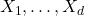be independent random variables taking values in a finite set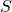, and let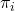be the probability distribution of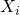.
Consider the chain on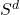which, at each move, picks a coordinateat random, and updates the value atwith an element ofchosen according to.
Show that for this chain, the spectral gap is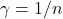.  Deduce the Efron-Stein inequality for these variables, see  wikipedia or Exercise 13.12 in the textbook for the statement.

3.* Show that Glauber dynamics for the Ising model has a transition matrixfor which all eigenvalues are non-negative.
Hint:  Show that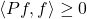for all functions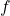on the state space.

4.* Assume the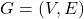is a graph,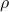is the graph distance on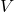, andis an irreducible transition matrix on.  Let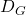be the diameter ofwith respect to this metric, and suppose that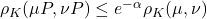for all choices of probability measureson.
Show that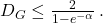## Reference

 Glasner, Shmuel.
Almost periodic sets and measures on the torus.
Israel J. Math. 32 (1979), no. 2-3, 161–-172.

 Berend, Daniel and Peres, Yuval.
Asymptotically dense dilations of sets on the circle.
J. London Math. Soc. (2) 47 (1993), no. 1, 1–-17.

 N. Alon and Y. Peres, "Uniform dilations'', Geometric and Functional Analysis, vol. 2, No. 1 (1992), 1–28.

 Nair, R. and Velani, S. L.
Glasner sets and polynomials in primes.
Proc. Amer. Math. Soc. 126 (1998), no. 10, 2835–-2840.